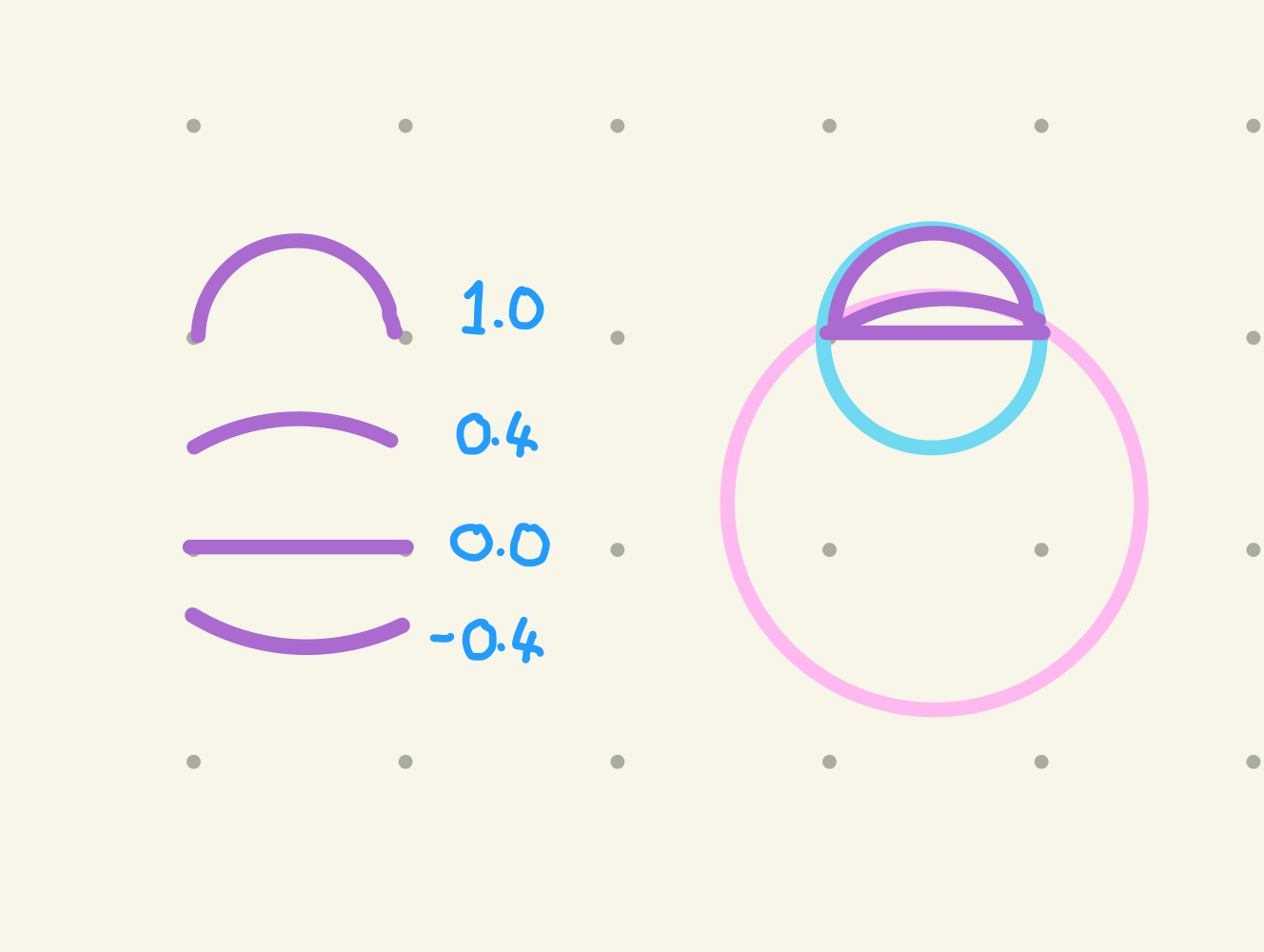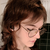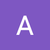# Line Curviness• updated
• DeclinedI am currently creating curved lines by using a small section of a disc, instead of a regular Line. It would be fantastic if this  could be built into the line inspector.

I'm using this technique to make flowers, but it's very difficult to setup manually, and the maths is tricky for me.

Reporting a bug? please specify Unity version:
Reporting a bug? please specify Shapes version:
Reporting a bug? please specify Render Pipeline:
Pinned repliesFreya Holmér
• Declined

I think this would be too specific of a feature to have on the line and use an extra parameter along with the specialized circular math needed in the shader, especially since it's essentially no longer a line as well as something that can be done with the arc already as is

If you want the math for it though, this might help! (requires my math library Mathfs)

`using Shapes;using UnityEngine;public class LineCurviness : MonoBehaviour {   public Vector2 start;   public Vector2 end;   [Range( -1f, 1f )] public float curviness = 0f;   void OnDrawGizmos() {      if( Mathf.Abs( curviness ) > 0.001f ) {                  // Mathfs         Vector2 normalOffset = ( end - start ).Rotate90CW() * curviness * 0.5f;         Vector2 lineCenter = ( start + end ) / 2;         Vector2 thirdPoint = lineCenter + normalOffset;         ( Vector2 center, float radius ) = Mathfs.Triangle.Circumcircle( start, end, thirdPoint );         Vector2 centerToStart = start - center;         Vector2 centerToEnd = end - center;         float angleBetween = Vector2.Angle( centerToStart, centerToEnd ) * Mathf.Deg2Rad;         float angToStart = centerToStart.Angle();         // apply         Disc disc = GetComponent<Disc>();         disc.transform.position = center;         disc.Radius = radius;         disc.AngRadiansStart = angToStart;         disc.AngRadiansEnd = angToStart + angleBetween * Mathf.Sign( curviness );      } else {         // line, not an arc      }         }}`
Replies 5Freya Holmér
• Declined

I think this would be too specific of a feature to have on the line and use an extra parameter along with the specialized circular math needed in the shader, especially since it's essentially no longer a line as well as something that can be done with the arc already as is

If you want the math for it though, this might help! (requires my math library Mathfs)

`using Shapes;using UnityEngine;public class LineCurviness : MonoBehaviour {   public Vector2 start;   public Vector2 end;   [Range( -1f, 1f )] public float curviness = 0f;   void OnDrawGizmos() {      if( Mathf.Abs( curviness ) > 0.001f ) {                  // Mathfs         Vector2 normalOffset = ( end - start ).Rotate90CW() * curviness * 0.5f;         Vector2 lineCenter = ( start + end ) / 2;         Vector2 thirdPoint = lineCenter + normalOffset;         ( Vector2 center, float radius ) = Mathfs.Triangle.Circumcircle( start, end, thirdPoint );         Vector2 centerToStart = start - center;         Vector2 centerToEnd = end - center;         float angleBetween = Vector2.Angle( centerToStart, centerToEnd ) * Mathf.Deg2Rad;         float angToStart = centerToStart.Angle();         // apply         Disc disc = GetComponent<Disc>();         disc.transform.position = center;         disc.Radius = radius;         disc.AngRadiansStart = angToStart;         disc.AngRadiansEnd = angToStart + angleBetween * Mathf.Sign( curviness );      } else {         // line, not an arc      }         }}`hayden scott-baron

I totally understand! Thanks for considering it, and wow - thanks for your script!You turned my frown upside down. :)
Thanks for making such a fantastic system!Anton Orlov

Years passed and now

`Mathfs.Triangle.Circumcircle`

no longer exists.

Could you help how to solve this problem with current version of Mathfs?

Thanks.Freya Holmér
Quote from Anton Orlov

Years passed and now

`Mathfs.Triangle.Circumcircle`

no longer exists.

Could you help how to solve this problem with current version of Mathfs?

Thanks.Anton Orlov

For anyone who need this here's the code:

```using Shapes;
using UnityEngine;
using Freya;

public class LineCurviness : MonoBehaviour {

public Vector2 start;
public Vector2 end;

[Range( -1f, 1f )] public float curviness = 0f;

void OnDrawGizmos() {
if( Mathf.Abs( curviness ) > 0.001f ) {

// Mathfs
Vector2 normalOffset = ( end - start ).Rotate90CW() * curviness * 0.5f;
Vector2 lineCenter = ( start + end ) / 2;
Vector2 thirdPoint = lineCenter + normalOffset;

Circle2D.FromThreePoints( start, end, thirdPoint, out Circle2D circle );
Vector2 centerToStart = start - circle.center;
Vector2 centerToEnd = end - circle.center;
float angleBetween = Vector2.Angle( centerToStart, centerToEnd ) * Mathf.Deg2Rad;
float angToStart = centerToStart.Angle();

// apply
Disc disc = GetComponent<disc>();
disc.transform.position = circle.center;